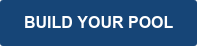Perhaps you are in the process of measuring your swimming pool, have come across the corners, and you're not sure how to measure them.  Or perhaps you're getting ready to measure your swimming pool and just want to research how to do it.  Either way, this short guide is about how to properly measure the corners and determine the corner radius of your inground swimming pool.There are several ways to measure and check your corner radius measurements. We will be illustrating the most common method, "Squaring the Corners".  We always recommend starting with this measurement method as it is the easiest.  In this method, you square the corners to find the radius.

To begin, follow an imaginary line past both sides of the radius until those lines intersect. See the photo for reference.

Once you have the corner squared, you then measure from the start of the radius to the point where the two imaginary lines intersect. This is your pool's corner radius measurement.

To double-check the corner radius measurement from above, you can use the length of the straight walls of the pool.  Basic mathematics will tell us that the Straight Wall + (Radius x 2) must equal the total width and length of your swimming pool.

For example; You have a 16' x 32' Rectangle Pool with 2' radius corners.  The long side measures 32'.  Since this pool has 2' radius corners, then the math to check the corner radius would be 32' - 2'- 2' = 28'.  So 28' would be the measurement of the long wall between the corners.  The short side math would be 16' - 2' - 2' = 12'.  So 12' would be the measurement of the short wall between the corners.  If the measurement you calculated for the straight section of wall between the corners is not correct, you will need to re-measure the radius to confirm it is correct. See the photo below for an example.Are you still nervous that you may have measured wrong?

No problem, there are a few more ways to double check the radius measurement. In the photo below, we listed the most common radius corners. The photo provides two additional measurements you can use to check your radius corner measurements.

To clarify some of the terms in the photo; The perimeter of the radius is the arc of the radius. You will need a flexible tape measure to get this measurement and will simply measure the total distance of the arc. The chord is a straight line connecting two points, the start of the radius on both sides.We can help here too.  Let's go over how to calculate both the Perimeter of the Radius and the Chord of the Radius.

To calculate the perimeter of a radius, multiply your radius measurement in inches times 1.5705 to give you the approximate perimeter measurement. For example; a 2'-6" radius converted to inches is 30".  So the perimeter measurement of a 2'6" radius would be 30" x 1.5705 = 47.115".

To calculate the chord of a radius, multiply the radius measurement in inches times 1.4142. For example; a 2' radius converted to inches is 24".  So the chord of a 2' radius would be 24" x 1.4142 = 33.9408.  *As shown in the photo above, you may round this measurement to nearest hundredth of inch 33.94".

### Filed Under:

Repairs, Tips, How-To, Inground Pools, Liners, Building